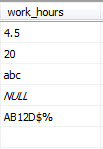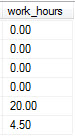# SQL Server – How to convert varchar to decimal

— This SQL reads in a varchar(10) field called work_hours that could have anything in it. It should be numeric represented as decimal.

— The CASE statement will produce either 0.00, or (if it is numeric) whatever is in the work_hours field.

— The is_numeric column is for analytical purposes and shows that the value of 1 means it was numeric and 0 when it was not numeric.

```SELECT column1, column2,
--ISNUMERIC(work_hours) As is_numeric,
CASE
WHEN ISNUMERIC(work_hours) = 0 THEN 0
WHEN work_hours LIKE '%[^-+ 0-9]%' THEN 0
WHEN isnumeric(CAST(CAST(work_hours as varchar(10)) as decimal(10,2))) = 1 then CAST(CAST(work_hours as varchar(10)) as decimal(10,2))
WHEN CAST(work_hours AS NUMERIC(38, 0))
NOT BETWEEN -2147483648. AND 2147483647. THEN 0
ELSE
1
END AS work_hours

FROM table
WHERE column1 = 'doe'```

If I had six rows of data where the work_hours had the following values:Then this SQL will produce the results as follows:Posted on Categories SQL Server